The acceleration-time graph of a body is shown below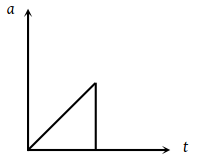The most probable velocity-time graph of the body is

 (1)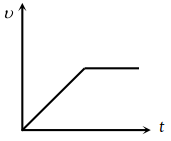(2)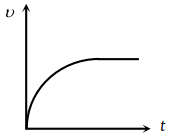(3)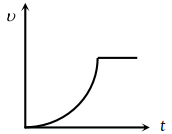(4)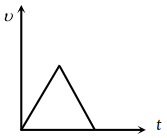Concept Videos :-

#20-Graph x vs t
#21- v vs t and a vs t graph
#22-Solved-Examples-12
#23-Solved-Examples-13
#24-Solved-Examples-14
#25-Solved-Examples-15

Concept Questions :-

Graphs

(3) From given $a-t$ graph it is clear that acceleration is increasing at constant rate

$\frac{da}{dt}=k$ (constant) ⇒ $a=kt$ (by integration)

$\frac{dv}{dt}=kt$$dv=ktdt$

${\int }_{}^{}dv=k{\int }_{}^{}tdt$$v=\frac{k{t}^{2}}{2}$

i.e. v is dependent on time parabolically and parabola is symmetric about v-axis.

and suddenly acceleration becomes zero. i.e. velocity becomes constant.

Hence (3) is most probable graph.

Difficulty Level:

• 22%
• 18%
• 53%
• 9%